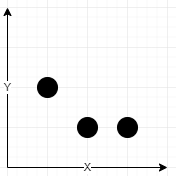# 网络信号最好的坐标

## 1620. 网络信号最好的坐标 (Medium)

• 坐标 `(x1, y1)` 字典序比另一个坐标 `(x2, y2)` 小：要么 `x1 < x2` ，要么 `x1 == x2` 且 `y1 < y2` 。
• `⌊val⌋` 表示小于等于 `val` 的最大整数（向下取整函数）。```输入：towers = [[1,2,5],[2,1,7],[3,1,9]], radius = 2

- 塔 (2, 1) 强度参数为 7 ，在该点强度为 ⌊7 / (1 + sqrt(0)⌋ = ⌊7⌋ = 7
- 塔 (1, 2) 强度参数为 5 ，在该点强度为 ⌊5 / (1 + sqrt(2)⌋ = ⌊2.07⌋ = 2
- 塔 (3, 1) 强度参数为 9 ，在该点强度为 ⌊9 / (1 + sqrt(1)⌋ = ⌊4.5⌋ = 4

```输入：towers = [[23,11,21]], radius = 9

```

```输入：towers = [[1,2,13],[2,1,7],[0,1,9]], radius = 2

```

```输入：towers = [[2,1,9],[0,1,9]], radius = 2

```

• `1 <= towers.length <= 50`
• `towers[i].length == 3`
• `0 <= xi, yi, qi <= 50`
• `1 <= radius <= 50`

[贪心算法]